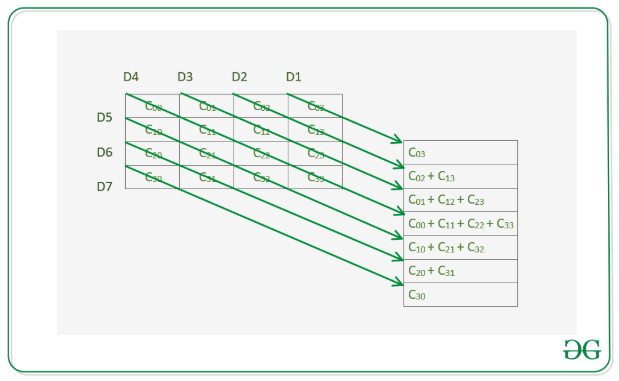# Maximum sum of elements in a diagonal parallel to the main diagonal of a given Matrix

• Difficulty Level : Medium
• Last Updated : 17 Mar, 2021

Give a square matrix mat[][] of dimensions N * N, the task is to find the maximum sum of elements present in the given matrix along the diagonals which are parallel to the main diagonal. Below is the image of the same.Examples:

Input: mat[][] = {{1, 2, 5, 7}, {2, 6, 7, 3}, {12, 3, 2, 4}, {3, 6, 9, 4}}
Output: 18
Explanation:
Sum of elements present in the diagonal having cells (2, 0) and (3, 1) is 12 + 6 = 18 which is maximum among all diagonals.

Input: mat[][] = {{5, 2, 5, 7}, {2, 5, 7, 3}, {12, 3, 5, 4}, {3, 6, 9, 5}}
Output: 18
Explanation:
Sum of elements present in the main diagonal having cells (0, 0), (1, 1), (2, 2) and (3, 3) is 5 + 5 + 5 + 5 = 20 which is maximum among all diagonals.

Approach: The idea is to traverse cells of each diagonal that is parallel to the main diagonal and observe that for any diagonal above the main diagonal starting at cell (x, y), it’s corresponding diagonal that is below the main diagonal will start at cell (y, x). For each diagonal, starting at cell (x, y) all its elements will be on cells (x + k, y + k) where 0 <= x + k, y + k < N. Follow the below steps to solve the problem:

• Initialize a variable maxSum with 0 which will store the maximum diagonal sum.
• Traverse the columns of 0th row from i over the range [0, N – 1].
• Initialize variables sum1 and sum2 which will store the diagonal sums starting from the cell (row, col) and from the cell (col, row) respectively where r is 0 and c is col.
• Increment both row and c by 1. Add mat[row][col] to sum1 and mat[col][row] to sum2 while row and col are smaller than N. Finally, update maxSum to store the maximum of maxSum, sum1, and sum2.
• After traversing the given matrix, print the value maxSum as the maximum sum.

Below is the implementation of the above approach:

## C++

 `// C++ program for the above approach` `#include ``using` `namespace` `std;` `// Function to return maximum diagonal``// sum that are parallel to main diagonal``int` `maxDiagonalSum(vector > arr, ``int` `N)``{``    ``// Initialize maxSum``    ``int` `maxSum = 0;` `    ``// Traverse through the columns``    ``for` `(``int` `i = 0; i < N; i++) {` `        ``// Initialize r and c``        ``int` `row = 0, col = i;` `        ``// Diagonal sums``        ``int` `sum1 = 0, sum2 = 0;``        ``while` `(col < N && row < N) {``            ``sum1 += arr[row][col];``            ``sum2 += arr[col][row];``            ``row++;``            ``col++;``        ``}` `        ``// Update maxSum with``        ``// the maximum sum``        ``maxSum = max({ sum1, maxSum, sum2 });``    ``}` `    ``// Return the maxSum``    ``return` `maxSum;``}` `// Driver Code``int` `main()``{``    ``// Given matrix mat[][]``    ``vector > mat``        ``= { { 1, 2, 5, 7 },``            ``{ 2, 6, 7, 3 },``            ``{ 12, 3, 2, 4 },``            ``{ 3, 6, 9, 4 } };``    ``int` `N = mat.size();` `    ``// Function Call``    ``cout << maxDiagonalSum(mat, N);` `    ``return` `0;``}`

## Java

 `// Java program for the above approach``import` `java.io.*;` `class` `GFG{``  ` `// Function to return maximum diagonal``// sum that are parallel to main diagonal``static` `int` `maxDiagonalSum(``int` `arr[][], ``int` `N)``{``    ` `    ``// Initialize maxSum``    ``int` `maxSum = ``0``;` `    ``// Traverse through the columns``    ``for``(``int` `i = ``0``; i < N; i++)``    ``{``        ` `        ``// Initialize r and c``        ``int` `row = ``0``, col = i;` `        ``// Diagonal sums``        ``int` `sum1 = ``0``, sum2 = ``0``;``        ``while` `(col < N && row < N)``        ``{``            ``sum1 += arr[row][col];``            ``sum2 += arr[col][row];``            ``row++;``            ``col++;``        ``}` `        ``// Update maxSum with``        ``// the maximum sum``        ``maxSum = Math.max(maxSum,``                          ``Math.max(sum1,``                                   ``sum2));``    ``}` `    ``// Return the maxSum``    ``return` `maxSum;``}` `// Driver code``public` `static` `void` `main (String[] args)``{``    ` `    ``// Given matrix mat[][]``    ``int` `mat[][] = { { ``1``, ``2``, ``5``, ``7` `},``                    ``{ ``2``, ``6``, ``7``, ``3` `},``                    ``{ ``12``, ``3``, ``2``, ``4` `},``                    ``{ ``3``, ``6``, ``9``, ``4` `} };``    ``int` `N = mat.length;` `    ``// Function Call``    ``System.out.println(maxDiagonalSum(mat, N));``}``}` `// This code is contributed by math_lover`

## Python3

 `# Python3 program for the above approach` `# Function to return maximum diagonal``# sum that are parallel to main diagonal``def` `maxDiagonalSum(arr, N):``    ` `    ``# Initialize maxSum``    ``maxSum ``=` `0` `    ``# Traverse through the columns``    ``for` `i ``in` `range``(N):``        ` `        ``# Initialize r and c``        ``row ``=` `0``        ``col ``=` `i` `        ``# Diagonal sums``        ``sum1 ``=` `0``        ``sum2 ``=` `0``        ` `        ``while` `col < N ``and` `row < N:``            ``sum1 ``+``=` `arr[row][col]``            ``sum2 ``+``=` `arr[col][row]``            ``row ``+``=` `1``            ``col ``+``=` `1` `        ``# Update maxSum with``        ``# the maximum sum``        ``maxSum ``=` `max``([ sum1, maxSum, sum2])` `    ``# Return the maxSum``    ``return` `maxSum` `# Driver Code``if` `__name__ ``=``=` `'__main__'``:``    ` `    ``# Given matrix mat[][]``    ``mat ``=` `[ [ ``1``, ``2``, ``5``, ``7` `],``            ``[ ``2``, ``6``, ``7``, ``3` `],``            ``[ ``12``, ``3``, ``2``, ``4` `],``            ``[ ``3``, ``6``, ``9``, ``4` `] ]` `    ``N ``=` `len``(mat)` `    ``# Function Call``    ``print``(maxDiagonalSum(mat, N))` `# This code is contributed by mohit kumar 29`

## C#

 `// C# program for the``// above approach``using` `System;``class` `GFG{``  ` `// Function to return maximum``// diagonal sum that are parallel``// to main diagonal``static` `int` `maxDiagonalSum(``int` `[,]arr,``                          ``int` `N)``{   ``  ``// Initialize maxSum``  ``int` `maxSum = 0;` `  ``// Traverse through the``  ``// columns``  ``for``(``int` `i = 0; i < N; i++)``  ``{``    ``// Initialize r and c``    ``int` `row = 0, col = i;` `    ``// Diagonal sums``    ``int` `sum1 = 0, sum2 = 0;``    ``while` `(col < N && row < N)``    ``{``      ``sum1 += arr[row,col];``      ``sum2 += arr[col,row];``      ``row++;``      ``col++;``    ``}` `    ``// Update maxSum with``    ``// the maximum sum``    ``maxSum = Math.Max(maxSum,``             ``Math.Max(sum1,``                      ``sum2));``  ``}` `  ``// Return the maxSum``  ``return` `maxSum;``}` `// Driver code``public` `static` `void` `Main(String[] args)``{   ``  ``// Given matrix [,]mat``  ``int` `[,]mat = {{1, 2, 5, 7},``                ``{2, 6, 7, 3},``                ``{12, 3, 2, 4},``                ``{3, 6, 9, 4}};``  ``int` `N = mat.GetLength(0);` `  ``// Function Call``  ``Console.WriteLine(maxDiagonalSum(mat, N));``}``}` `// This code is contributed by gauravrajput1`

## Javascript

 ``

Output

`18`

Time Complexity: O(N2)
Auxiliary Space: O(N2)

My Personal Notes arrow_drop_up